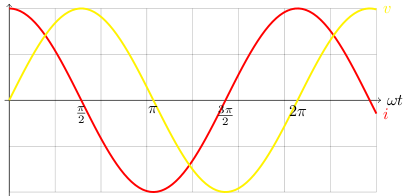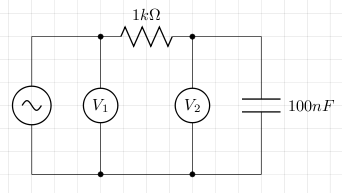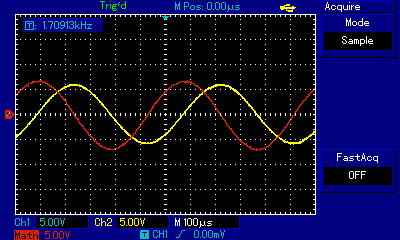# Capacitive Reactance

Capacitive reactance is the opposition to alternating current produced by capacitance. The magnitude of reactance depends upon the rate of change of voltage.

Consider a capacitor connected to an alternating voltage source. If i, v, and q are the instantaneous current, voltage and charge respectively, then:

```C=Q/V
C=q/v
q=Cv
dq/dt=Cdv/dt
i=Cdv/dt
```

The instantaneous current is at its maximum when the rate of change of voltage is also at its maximum. When the capacitor is fully charged, the voltage across it is at its maximum and the current is zero. The graph below shows the relationship between current and voltage across the capacitor. Here ω is the angular frequency. Note that the current leads the voltage by π/2.Let V0 be the maximum voltage, and I0 be the maximum current:

```v=V0sin(ωt)
i=C(dV0sin(ωt))/dt
i=ωCV0cos(ωt)
=ωCV0sin(ωt + π/2)
I0=ωCV0   [i=I0 when sin(ωt + π/2)=1]
```

Capacitive reactance is measured in ohms and written XC. It is calculated as follows

```XC=V0/I0
=V0/ωCV0
=1/ωC
```

At high frequencies the current will not have a chance to reach zero before the current alternates which means the reactance will be less than that at lower frequencies where the current will have a chance to reach zero.

### Practical Demonstration

Consider the following circuit. V1 is measured by channel 1 on an oscilloscope, and V2 is measured by channel 2. The difference between them (V1 - V2) should give us the voltage across the resistor and according to Ohm's law, this is proportional to the current (in our case we just divide by 1000).The screen shot from the oscilloscope shows the voltage across the resistor in red and the voltage across the capacitor in yellow. We see that the current leads the voltage by π/2 and this is in agreement with the graph above.## References

Fischer-Cripps. A.C., The Electronics Companion. Institute of Physics, 2005.